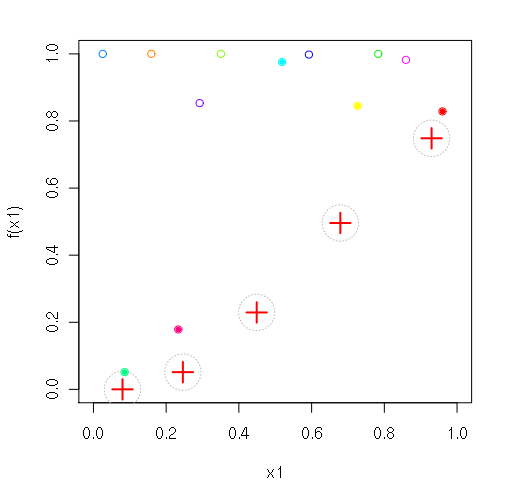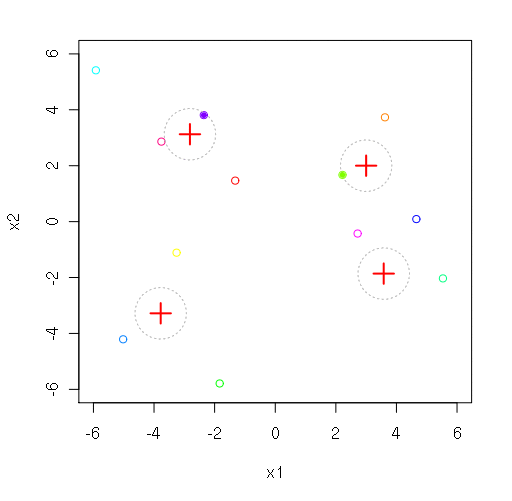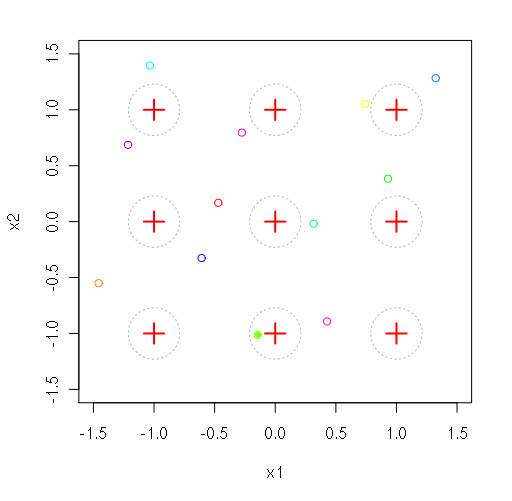Repository for Ideas & Research
Open Source GIS, Hydrologic Modeling, Optimization

# Isolated-speciation-based particle swarm optimization (ISPSO)

Since December 18, 2011 by Huidae Cho

The ispso.R file is an R script that implements the ISPSO (Isolated-Speciation-based PSO) algorithm introduced by Cho et al. (2011). The author’s version of Cho et al. (2011) is available here. ISPSO aims to find global and local minima of the objective function. This R script file is distributed under the GNU Public License. If you have questions about this software, leave a comment here.

ISPSO was successfully applied to stochastic rainfall generation (Kim et al. 2013a, Kim et al. 2013b, Kim et al. 2013c, Kim et al. 2016), storm tracking (Cho et al. 2013), uncertainty analysis (Cho and Olivera 2014, Cho et al. 2014), climate change studies (Heo et al. 2015a, Heo et al. 2015b), and floodway study (Cho et al., 2018).

Please cite this software as “Huidae Cho, Dongkyun Kim, Francisco Olivera, Seth D. Guikema, 2011. Enhanced Speciation in Particle Swarm Optimization for Multi-Modal Problems. European Journal of Operational Research 213 (1), 15–23.”

## 1   1-dimensional function

$$F4(x)=1-\exp\left(-2\log(2)\cdot\left(\frac{x-0.08}{0.854}\right)^2\right)\cdot\sin^6\left(5\pi(x^{3/4}-0.05)\right)$$## 2   Himmelblau function

$$F5(x_1, x_2)=(x_1^2+x_2-11)^2+(x_1+x_2^2-7)^2$$## 3   Rastrigin function

$$F6(\vec{x})=\sum_{i=1}^D\left[x_i^2-10\cos(2\pi x_i)+10\right]$$## 4   Griewank function

$$F7(\vec{x})=\frac{1}{4000}\sum_{i=1}^dx_i^2-\prod_{i=1}^d\cos\left(\frac{x_i}{\sqrt{i}}\right)+1$$